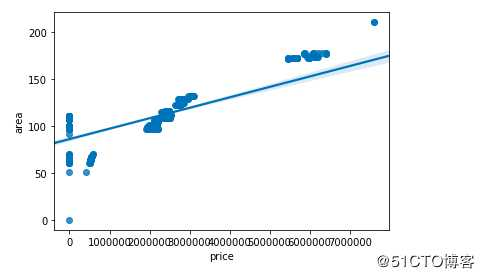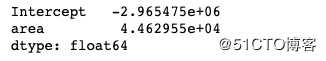• 来自烟水暖的学习笔记回归分析(Regression analysis)回归...回归分析的分类：1） 按自变量的个数，可以分为一元回归，多元回归2）按变量相关性的形状（回归线）是否为直线型，可分为线性回归，非线性回归。下面，...
来自烟水暖的学习笔记回归分析(Regression analysis)回归分析(Regression analysis)，是研究因变量与自变量之间相关性的一种数学方法，并将相关性量化,即得到回归方程。我们可以通过回归方程（回归预测模型）来预测因变量的变化。回归分析的分类：1） 按自变量的个数，可以分为一元回归，多元回归2）按变量相关性的形状（回归线）是否为直线型，可分为线性回归，非线性回归。下面，我们将通过python工具来理解最简单的回归分析，即一元线性回归，并了解回归分析的步骤。想要更多了解回归分析包含的内容，可参考下面内容烟水暖：详细解读Excel回归分析：价格与需求的相关性​zhuanlan.zhihu.com一元线性回归顾名思义，就是只包含一个自变量，且与因变量的关系是呈线性的回归分析，其回归方程（回归模型）可用下面的方程表示：y=bx+a （其中:y为因变量，x为自变量，b为回归系数或斜率，a为截距）简单实例我们将通过分析产品A的单价与销量之间的关系，来预测当产品A的定价为x，市场的需求量y将会是多少，这样我们就能知道该向供应商订购多少产品A。数据如下：确定变量，导入数据2. 探索性数据分析，绘制散点图，观察变量之间关系及趋势变化，确定回归类型#绘制散点图，观察Price,demand的相关性由图可见，Price与Demand具有较强的负相关性，即Price增加，Demand反而减少。我们也可通过下面函数观察Price与Demand的相关系数（相关系数R是描述变量之间的相关性，范围在[-1,1]之间，R>0，则为正相关，R<0，则为负相关；R的绝对值越接近于1，相关性越强，反之，相关性越弱）3. 建立回归模型，并进行模型训练在统计学中，一般我们是通过“最小二乘法”直线拟合来执行线性回归分析。4. 检验模型的拟合程度查看模型的判断系数，判断其拟合度。判断系数为0.8679788013877346，可见模型的拟合程度还是较高的。下面将拟合回归线放入散点图，直观的感受模型的拟合程度:最后查看回归方程的内容:模型包含的内容：参数：fit_intercept： 默认为true,是否计算截距normalize： 是否将数据归一化copy_X：是否复制x的值，默认是复制，否则覆盖原来x的值n_jobs：计算模型使用的作业数，也叫核数属性：coef_:斜率intercept_:截距方法：fit() 拟合线性模型get_params() 获取估计量的参数,即返回上面四个参数的内容predict() 使用模型预测结果score()  返回判定系数 ^2,判定系数描述的是回归方程的拟合优度，值越接近于1,拟合度越高set_params()设置估计量的参数的内容这里，我们只需要拿到下面内容，即可知道回归模型的内容。即Price与Demand呈负相关性，且可用 y=-0.895x+10.128来描述其关系。接下来，我们便可用这个模型来预测需求。5. 使用回归模型预测通过模型的预测方法，我们可预测当产品A的价格为8，或8.2，对应的需求为2.96，2.78.总结通过上面的学习，我们可用了解到最简单的线性回归分析，理解其如何帮我们来分析Price和Demand之间的关系，并由此预测Demand。
展开全文• 线性回归模型建模步骤 （一元线性回归、多元线性回归）
• 一元线性回归模型 线性回归模型是数据科学领域最简单的模型，很多复杂的模型 （如多项式回归、逻辑回归、SVM） 都是建立在线性回归模型的基础上的，或者是从其中能找到线性回归模型的影子。最简单的线性回归模型就是...
本文主要介绍线性回归模型，该模型主要应用于监督学习中目标变量是连续数值型的场景。
一元线性回归模型
线性回归模型是数据科学领域最简单的模型，很多复杂的模型 （如多项式回归、逻辑回归、SVM） 都是建立在线性回归模型的基础上的，或者是从其中能找到线性回归模型的影子。最简单的线性回归模型就是一元线性回归模型，其模型的形式就是：
$y=ax+b \tag{1}$
由式(1)可知，线性回归是针对连续数值型变量的模型，且样本只有一个特征，即只有一个自变量。所谓线性，是指方程是线性的，也就是在寻找一条直线来拟合数据；所谓回归，是指用方程来模拟变量之间是如何关联的。线性回归就是要找一条直线，并且让这条直线尽可能地拟合训练样本中的数据点。
虽然我们可以找到一条拟合训练集的直线，但是同一训练样本可以拟合出很多条直线，我们如何判断那条直线是最适合的？这就引出了如何评价模型结果的好坏，又通过什么样的方式来判断？接下来我们通过损失函数来了解线性回归的评价标准。
损失函数
为了衡量模型的效果，很自然的就会想到衡量预测值与真实值之间的差别。假设预测值为f(x)，真实值为y，样本的数量为n，那么就有：
$\sum_{i=1}^n(f(x_i)-y_i)^2 \tag{2}$
也就是每一个样本的预测值与真实值差的平方和，取平方是为了消除负数的影响。
式(2)就是均方误差(MSE, Mean Squared Error)，它对应了常用的欧氏距离（也就是计算两点之间距离）。均方误差是机器学习中回归问题经常使用的评价方式，在机器学习中称之为损失函数。可以看到一个公式在不同的地方会有不同的名字，很容易搞混，但我们只要理解其中的基本思想就会很清楚了。
所以，现在我们知道了损失函数是衡量回归模型误差的函数，也就是我们要的“直线”的评价标准。这个函数的值越小，说明直线越能拟合我们的数据。
得到损失函数后，又如何求得使损失函数最小的a和b呢，接下来我们通过最小二乘法来获得问题的解。
最小二乘法
回归方程里，求得特征对应的最佳回归系数的方法是最小化误差的平方和，也就是损失函数。
假设式(2)的值为S，将式(1)代入式(2)可得：
$S =\sum_{i=1}^n(f(x_i)-ax_i-b)^2 \tag{3}$
在xi和yi已知的前提下，式(3)是a和b的二次函数。由微积分的知识可知，要求得a和b的最小值分别对a和b求偏导，当两者的偏导数为0时取得最小值。如下所示：
$\frac {\partial S } {\partial a }=2\sum_{i=1}^n(y_i - ax_i-b)x_i = 0 \tag{4}$
$\frac{\partial S}{\partial b} = 2\sum_{i=1}^n(y_i-ax_i-b)=0 \tag{5}$
将xi和yi代入(4)和(5)式中就可以得到a和b的最小值。
总结
本文简单介绍了一元线性回归模型及其损失函数，并通过最小二乘法求得线性方程的最小参数。
参考资料
模型之母：简单线性回归&最小二乘法
用人话讲明白线性回归LinearRegression
机器学习眼中的线性回归


展开全文损失函数 机器学习 最小二乘法
• 接下来，建模： import statsmodels.api as sm fit=sm.formula.ols(‘price~area‘,data=data).fit() print(fit.params) 这就是他们的参数，得到一个一元一次线性关系： price=-2.965475+4.462955*area 用python解...
import pandas as pdimport numpy as npimport seaborn as snsdata=pd.read_excel(r‘/Users/fangluping/Desktop/房源销售影响因素/望潮府.xlsx‘，usecols=[‘price‘,‘area‘])sns.regplot(‘price‘,‘area‘,data=data)样本量太少，大致认为它是吧。接下来，建模：import statsmodels.api as smfit=sm.formula.ols(‘price~area‘,data=data).fit()print(fit.params)这就是他们的参数，得到一个一元一次线性关系：price=-2.965475+4.462955*area用python解数学题是不是很简单，秒解。原文地址：https://blog.51cto.com/14534896/2488438
展开全文• 一元线性回归模型很简单y1=ax+b+ε，y1为实际值，ε为正态的误差。y2=ax+b，y2为预测值。ε=y1-y2。def model(a,b,x):# x is vector，a and b are the common number.return a*x+b这里将整组数据的预测结果方差作为...
一元线性回归模型很简单y1=ax+b+ε，y1为实际值，ε为正态的误差。y2=ax+b，y2为预测值。ε=y1-y2。def model(a,b,x):# x is vector，a and b are the common number.return a*x+b这里将整组数据的预测结果方差作为损失函数。J(a,b)=sum((y1-y2)^2)/ndef cost(a,b,x,y):# x is argu, y is actual result.n=len(x)return np.square(y-a*x-b).sum()/n优化函数则进行使损失函数，即方差最小的方向进行搜索a=a-theta*(∂J/∂a)b=b-theta*(∂J/∂b)这里的解释就是，对影响因素a或b求损失函数J的偏导，如果损失函数随着a或b增大而增大，我们就需要反方向搜索，使得损失函数变小。对于偏导的求取则看的别人的推导公式theta为搜索步长，影响速度和精度(我猜的，实际没有验证)def optimize(a,b,x,y):theta = 1e-1  # settle the step as 0.1n=len(x)y_hat = model(a,b,x)# compute forecast valuesda = ((y_hat-y)*x).sum()/ndb = (y_hat-y).sum()/na = a - theta*dab = b - theta*dbreturn a, b使用sklearn库，可以使用现成的线性回归模型import numpy as npfrom sklearn.linear_model import LinearRegressionimport matplotlib.pyplot as pltx = [1,2,4,5,6,6,7,9]x = np.reshape(x,newshape=(8,1))y =  [10,9,9,9,7,5,3,1]y = np.reshape(y,newshape=(8,1))# create an instance of LinearRegression modellr = LinearRegression()# train modellr.fit(x,y)lr.score(x,y)# compute y_haty_hat = lr.predict(x)# show the resultplt.scatter(x,y)plt.plot(x, y_hat)plt.show()
展开全文• -*-coding:utf-8-*-import numpy as npimport matplotlib.pyplot as plt2、定义线性回归模型def predict(x, a, b):y_predict = a * x + breturn y_predict一元线性回归的模型如下：公式1—一元线性回归模型损失函数...
• 问题制作一元材积表，不懂林学的可能不知道，如图，也就是构造材积和胸径间的关系，这里采用了python的一元线性回归方法（本人用spss做了幂函数非线性回归，效果最好）。Python方差分析导入库和数据from sklearn ...
• 一元线性回归模型显著性检验方法的改进一元线性回归模型显著性检验方法的改进一元线性回归模型显著性检验方法的改进一元线性回归模型显著性检验方法的改进
• 一元线性回归分析模型在家庭消费支出预测中的应用，高玉，周树民，介绍一元线性回归分析的基本概念和方法原理，并以2001年到2010年国民的城镇居民家庭人均支配收入（简称
• 基于修正的一元线性回归模型的企业利润预测研究，张琛，那宝贵，本文针对一元线性回归模型进行研究，用马尔可夫链状模型对一元线性回归模型进行修正和改进，使得修正后的一元线性回归模型更加的
• Jupyter nodebook工具做的简单的一元线性回归。将文件导入nodebook运行即可 数据摘自深入浅出数据分析这本书。这里用python3实现书中的R代码。有需要的学习python3进行数据分析的同学自行倒腾
• Matlab中实际上有多个函数可以实现回归分析的功能，如regress，polyfit，lsqcurvefit等。这里简单总结一下polyfit函数的用法：Matlab中实际上有多个函数可以实现回归分析的功能，如regress，polyfit，lsqcurvefit等...
• 简要：实际上计量模型估计和实现最好用的软件还是stata，这个软件无论在国内还是国外，经济学家们都是爱不释手。今天我们要介绍的是python中的一个较为强大的统计分析包(Statsmodels)，不过需要强调的是python因为...
• 之前的博文讲的是理论。现在需要用到成熟的库，来应用。 Science 科学的工具包 kit # sklearn 命名惯例： ...# LinearRegression 线性回归的类，我们可以使用该类实现线性回归。 # 底层就是使用...机器学习 sklearn 模型可视化
• """ 《深度学习与图像识别原理实践》 """ import numpy as np class SimpleLinearRegressionSelf... 初始化Simple Linear regression 模型 """ self.a_=None self.b_=None def fit(self,x_train,y_train): asser.python 机器学习
• 一元线性回归分析预测法模型，根据历史数据预测下期数据值
• 一元线性回归模型 参数估计（最小二乘法） 最小二乘法c++实现 导言 监督学习中，预测的变量是离散的，则称分类 otherwise 连续的变量称回归。 回归中只包含一个自变量和因变量，二者为线性关系，则为一元线性...
• 一元线性回归spss处理方法，可以了解SPSS对线性回归的处理内容
•最小二乘法
•matlab
• 线性回归分析学习笔记第一篇：一元线性回归 1.线性回归分析究竟是何方神圣？ 首先要弄懂什么是回归分析： 在大数据分析中，回归分析是一种预测性的建模技术，它研究的是因变量（目标）和自变量（预测器）之间的...机器学习...